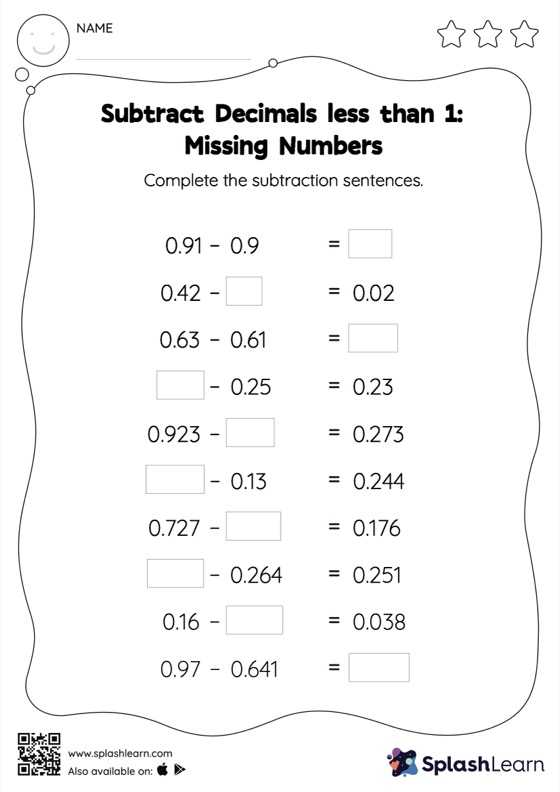# Subtract Decimals less than 1: Missing Numbers Worksheet

Home > Subtract Decimals less than 1: Missing NumbersThis worksheet challenges young mathematicians to hone their math skills by solving a set of problems on subtracting decimals less than 1. As the worksheet uses a horizontal format, it allows for more creativity when it comes to how the student solves the problem. In contrast, in the vertical format, students usually employ the standard method to solve.